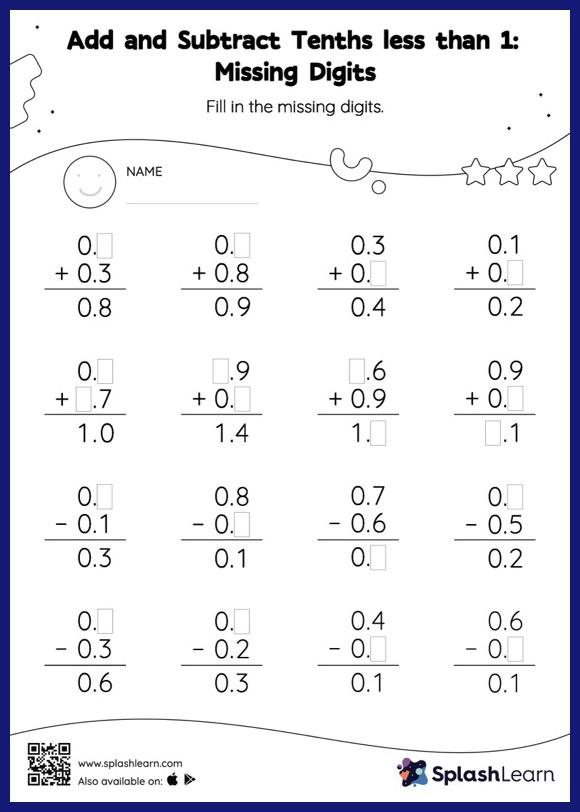# Add and Subtract Tenths less than 1: Missing Digits Worksheet

Home > Add and Subtract Tenths less than 1: Missing DigitsIn this worksheet, students add and subtract decimals by aligning the decimal points and using zero as a placeholder. They then use the relationship between addition and subtraction to find the missing number in the add and subtract tenths less than 1 worksheet. This worksheet is about practicing the column method in which numbers are written one on top of another. In this method, students use their place value understanding to solve the problems.